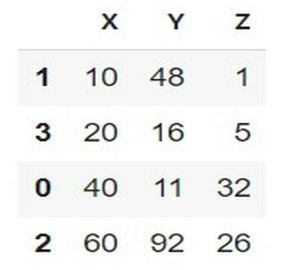# How to Sort a Pandas DataFrame based on column names or row index?

Many applications benefit from sorting pandas DataFrame by column names or row indexes. For example, to show how sales continue over time, we may sort a DataFrame of sales data by date. In Python, we have some built-in functions- DataFrame(), sort_index(), and, sort_values() that can be used to Sort a Pandas DataFrame based on column names or row index.

## Syntax

The following syntax is used in the examples −

DataFrame(var_name, colums= ['col1', 'col2', and so on], index= ['1', '2', and so on])


A DataFrame is a library of pandas modules and defines the 2D structure of different rows and columns.

sort_index()


The sort_index is sort the series by index labels. This method sorts the pandas dataframe

in ascending and descending order.

sort_index(axis = 1)


This sort_index accepts the parameter named axis = 1 that sort the column order. In other words, we can say that the axis = 1 specifies the columns.[Example 3]

sort_values(by=["col1","col2","col3"])


The sort_value method is defined by sorting the items or series in ascending order. The above representation accepts three columns as parameters to sort their item or series.

sort_values(by=["row1","row2","row2"])


The above representation accepts three rows to sort their item or series by using the technique of list datatype.

## Example 1

In the following example, we will start the program by importing the module named pandas. Take pd as its object reference. Then create the employee data using list comprehension and store it in the variable Emp. Then create an object DataFrame from a list of tuples using columns and rows and store it in the variable info. Next, mention the variable info and get the tabular structure of the data.

import pandas as pd
# List of Tuples
Emp = [('Arun', 24, 'Uttrakhand', 'Tester', 'Male'),
('Shyam', 23, 'West Bengal', 'SDE-1', 'Male'),
('Raghav', 37, 'Maharastra', 'SDE-3', 'Male'),
('Jayanti', 29, 'Kerala', 'Customer Support','Female')]
# Dataframe object from list of tuples using column and index
info = pd.DataFrame(Emp, columns =['Name', 'Age',
'Place', 'Designation','Gender'],
index =[ '105', '109', '110', '104'])
# Show the dataframe
info


## Output## Example 2

In the following example, the below code follows the sequence order of code by using the next terminal. Then use the built-in method sort_index() which will sort the row in ascending order and store it in the variable sort_idx. Finally, use the variable sort_idx to get the processed data according to the given code.

# sort the index row
sort_idx = info.sort_index()
sort_idx


## Output## Example 3

In the following example, the below code follows the sequence order of the above code by using the next terminal. Here we will implement the program based on column sorting. Then import pandas to start the program(not necessarily needed). Next, use the built-in method sort_index() that will sort the column in ascending order. Then simply write the variable named sort_col to get the result in one another form.

# sort the column
import pandas as pd
sort_col = info.sort_index(axis = 1)
sort_col


## Output## Example 4

In the following example, begin the program by importing the module named pandas that set the object reference as pd. Then use the dictionary datatype to set the three columns namely X, Y, and Z to store it in the variable col. Next, use the DataFrame of pandas module and store it in a new variable named df. Now sort the rows using the built-in method sort_values that follow the series or items in ascending order and store it in the variable sorted_df. Then simply write the sorted_df to get the tabular output as a result.

# Sort DataFrame rows based on multiple columns
import pandas as pd

# create the dictionary
col = {"X" : [40, 10, 60, 20], "Y":[11, 48, 92, 16], "Z":[32,1,26,5]}
df = pd.DataFrame(col)

#Mention the row for sorting
sorted_df=df.sort_values(by=["X","Y","Z"])
sorted_df


## Output## Example 5

In the following example, start the program by importing the module named pandas. Take an object reference named pd that will be later used in the built-in method sort_values. Then create the list that makes the data of three different items of rows namely P, Q, and, R and store it in the variable list1. Next, use the DataFrame of pandas module that accepts two parameters- list1(the previous variable name to use the data) and index( this parameter set the values of all columns by using an in-built method list). Moving ahead to use the built-in method named sort_values that accepts the following parameters −

by=['P','Q','R']: The keyword by set the number of rows i.e. P, Q, and, R.

axis = 1: identify the columns.

Finally, we are printing the result with the help of variable sorted_row.

# Sort Dataframe based on multiple rows
import pandas as pd
list1 = [(5,40,3,2),(11,4,12,6),(13,91,16,5)]
df = pd.DataFrame(list1, index=list('PQR'))
sorted_row = df.sort_values(by=['P','Q','R'],axis=1)
sorted_row


## Output## Conclusion

We discussed the different ways to sort the columns names or row indexes using Pandas Dataframe. The first example explains the simple tabular structure of rows and columns whereas the second and third examples follow the serial order to complete the meaningful representation of datasets. The fourth example uses the dictionary technique to create the data for multiple columns whereas the fifth example uses the list datatype to create the data for multiple rows and generated different outputs as a result.

Updated on: 17-Jul-2023

963 Views